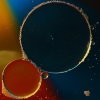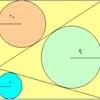# Search by Topic

#### Resources tagged with Regular polygons and circles similar to Over the Pole:

Filter by: Content type:
Age range:
Challenge level:

### There are 67 results

Broad Topics > Angles, Polygons, and Geometrical Proof > Regular polygons and circles### Circumnavigation

##### Age 14 to 16 Challenge Level:

The sides of a triangle are 25, 39 and 40 units of length. Find the diameter of the circumscribed circle.### Holly

##### Age 14 to 16 Challenge Level:

The ten arcs forming the edges of the "holly leaf" are all arcs of circles of radius 1 cm. Find the length of the perimeter of the holly leaf and the area of its surface.### 2D-3D

##### Age 16 to 18 Challenge Level:

Two circles of equal size intersect and the centre of each circle is on the circumference of the other. What is the area of the intersection? Now imagine that the diagram represents two spheres of. . . .### So Big

##### Age 16 to 18 Challenge Level:

One side of a triangle is divided into segments of length a and b by the inscribed circle, with radius r. Prove that the area is: abr(a+b)/ab-r^2### Logosquares

##### Age 16 to 18 Challenge Level:

Ten squares form regular rings either with adjacent or opposite vertices touching. Calculate the inner and outer radii of the rings that surround the squares.### Dodecawhat

##### Age 14 to 16 Challenge Level:

Follow instructions to fold sheets of A4 paper into pentagons and assemble them to form a dodecahedron. Calculate the error in the angle of the not perfectly regular pentagons you make.### The Dodecahedron

##### Age 16 to 18 Challenge Level:

What are the shortest distances between the centres of opposite faces of a regular solid dodecahedron on the surface and through the middle of the dodecahedron?### First Forward Into Logo 2: Polygons

##### Age 7 to 16 Challenge Level:

This is the second in a twelve part introduction to Logo for beginners. In this part you learn to draw polygons.### Like a Circle in a Spiral

##### Age 7 to 16 Challenge Level:

A cheap and simple toy with lots of mathematics. Can you interpret the images that are produced? Can you predict the pattern that will be produced using different wheels?### First Forward Into Logo 4: Circles

##### Age 7 to 16 Challenge Level:

Learn how to draw circles using Logo. Wait a minute! Are they really circles? If not what are they?### Ford Circles

##### Age 16 to 18 Challenge Level:

Can you find the link between these beautiful circle patterns and Farey Sequences?### Semi-square

##### Age 14 to 16 Challenge Level:

What is the ratio of the area of a square inscribed in a semicircle to the area of the square inscribed in the entire circle?### LOGO Challenge 12 - Concentric Circles

##### Age 11 to 16 Challenge Level:

Can you reproduce the design comprising a series of concentric circles? Test your understanding of the realtionship betwwn the circumference and diameter of a circle.### Quadarc

##### Age 14 to 16 Challenge Level:

Given a square ABCD of sides 10 cm, and using the corners as centres, construct four quadrants with radius 10 cm each inside the square. The four arcs intersect at P, Q, R and S. Find the. . . .### Circle Packing

##### Age 14 to 16 Challenge Level:

Equal circles can be arranged so that each circle touches four or six others. What percentage of the plane is covered by circles in each packing pattern? ...### Semi-detached

##### Age 14 to 16 Challenge Level:

A square of area 40 square cms is inscribed in a semicircle. Find the area of the square that could be inscribed in a circle of the same radius.### Squaring the Circle and Circling the Square

##### Age 14 to 16 Challenge Level:

If you continue the pattern, can you predict what each of the following areas will be? Try to explain your prediction.### Pegboard Quads

##### Age 14 to 16 Challenge Level:

Make five different quadrilaterals on a nine-point pegboard, without using the centre peg. Work out the angles in each quadrilateral you make. Now, what other relationships you can see?### Bicentric Quadrilaterals

##### Age 14 to 16 Challenge Level:

Investigate the properties of quadrilaterals which can be drawn with a circle just touching each side and another circle just touching each vertex.### Some(?) of the Parts

##### Age 14 to 16 Challenge Level:

A circle touches the lines OA, OB and AB where OA and OB are perpendicular. Show that the diameter of the circle is equal to the perimeter of the triangle### Get Cross

##### Age 14 to 16 Challenge Level:

A white cross is placed symmetrically in a red disc with the central square of side length sqrt 2 and the arms of the cross of length 1 unit. What is the area of the disc still showing?### Escriptions

##### Age 16 to 18 Challenge Level:

For any right-angled triangle find the radii of the three escribed circles touching the sides of the triangle externally.### Lunar Angles

##### Age 16 to 18 Challenge Level:

What is the sum of the angles of a triangle whose sides are circular arcs on a flat surface? What if the triangle is on the surface of a sphere?### Kissing

##### Age 16 to 18 Challenge Level:

Two perpendicular lines are tangential to two identical circles that touch. What is the largest circle that can be placed in between the two lines and the two circles and how would you construct it?### Orthogonal Circle

##### Age 16 to 18 Challenge Level:

Given any three non intersecting circles in the plane find another circle or straight line which cuts all three circles orthogonally.### Area I'n It

##### Age 16 to 18 Challenge Level:

Triangle ABC has altitudes h1, h2 and h3. The radius of the inscribed circle is r, while the radii of the escribed circles are r1, r2 and r3 respectively. Prove: 1/r = 1/h1 + 1/h2 + 1/h3 = 1/r1 +. . . .### Retracircles

##### Age 16 to 18 Challenge Level:

Four circles all touch each other and a circumscribing circle. Find the ratios of the radii and prove that joining 3 centres gives a 3-4-5 triangle.### LOGO Challenge 11 - More on Circles

##### Age 11 to 16 Challenge Level:

Thinking of circles as polygons with an infinite number of sides - but how does this help us with our understanding of the circumference of circle as pi x d? This challenge investigates. . . .### Crescents and Triangles

##### Age 14 to 16 Challenge Level:

Can you find a relationship between the area of the crescents and the area of the triangle?### LOGO Challenge 10 - Circles

##### Age 11 to 16 Challenge Level:

In LOGO circles can be described in terms of polygons with an infinite (in this case large number) of sides - investigate this definition further.### Tessellation Interactivity

##### Age 7 to 16 Challenge Level:

An environment that enables you to investigate tessellations of regular polygons### Arclets Explained

##### Age 11 to 16

This article gives an wonderful insight into students working on the Arclets problem that first appeared in the Sept 2002 edition of the NRICH website.### Circumspection

##### Age 14 to 16 Challenge Level:

M is any point on the line AB. Squares of side length AM and MB are constructed and their circumcircles intersect at P (and M). Prove that the lines AD and BE produced pass through P.### Three Four Five

##### Age 14 to 16 Challenge Level:

Two semi-circles (each of radius 1/2) touch each other, and a semi-circle of radius 1 touches both of them. Find the radius of the circle which touches all three semi-circles.### F'arc'tion

##### Age 14 to 16 Short Challenge Level:

At the corner of the cube circular arcs are drawn and the area enclosed shaded. What fraction of the surface area of the cube is shaded? Try working out the answer without recourse to pencil and. . . .### Tricircle

##### Age 14 to 16 Challenge Level:

The centre of the larger circle is at the midpoint of one side of an equilateral triangle and the circle touches the other two sides of the triangle. A smaller circle touches the larger circle and. . . .### Two Regular Polygons

##### Age 14 to 16 Challenge Level:

Two polygons fit together so that the exterior angle at each end of their shared side is 81 degrees. If both shapes now have to be regular could the angle still be 81 degrees?### From All Corners

##### Age 14 to 16 Challenge Level:

Straight lines are drawn from each corner of a square to the mid points of the opposite sides. Express the area of the octagon that is formed at the centre as a fraction of the area of the square.### Baby Circle

##### Age 16 to 18 Challenge Level:

A small circle fits between two touching circles so that all three circles touch each other and have a common tangent? What is the exact radius of the smallest circle?### LOGO Challenge 6 - Triangles and Stars

##### Age 11 to 16 Challenge Level:

Recreating the designs in this challenge requires you to break a problem down into manageable chunks and use the relationships between triangles and hexagons. An exercise in detail and elegance.### LOGO Challenge 9 - Overlapping Polygons

##### Age 7 to 16 Challenge Level:

This LOGO challenge starts by looking at 10-sided polygons then generalises the findings to any polygon, putting particular emphasis on external angles### Circles in Circles

##### Age 16 to 18 Challenge Level:

This pattern of six circles contains three unit circles. Work out the radii of the other three circles and the relationship between them.### Incircles Explained

##### Age 16 to 18

This article is about triangles in which the lengths of the sides and the radii of the inscribed circles are all whole numbers.### Curvy Areas

##### Age 14 to 16 Challenge Level:

Have a go at creating these images based on circles. What do you notice about the areas of the different sections?### LOGO Challenge 1 - Star Square

##### Age 7 to 16 Challenge Level:

Can you use LOGO to create this star pattern made from squares. Only basic LOGO knowledge needed.### Spokes

##### Age 16 to 18 Challenge Level:

Draw three equal line segments in a unit circle to divide the circle into four parts of equal area.### Sangaku

##### Age 16 to 18 Challenge Level:

The square ABCD is split into three triangles by the lines BP and CP. Find the radii of the three inscribed circles to these triangles as P moves on AD.### Gold Yet Again

##### Age 16 to 18 Challenge Level:

Nick Lord says "This problem encapsulates for me the best features of the NRICH collection."### Partly Circles

##### Age 14 to 16 Challenge Level:

What is the same and what is different about these circle questions? What connections can you make?### Efficient Packing

##### Age 14 to 16 Challenge Level:

How efficiently can you pack together disks?